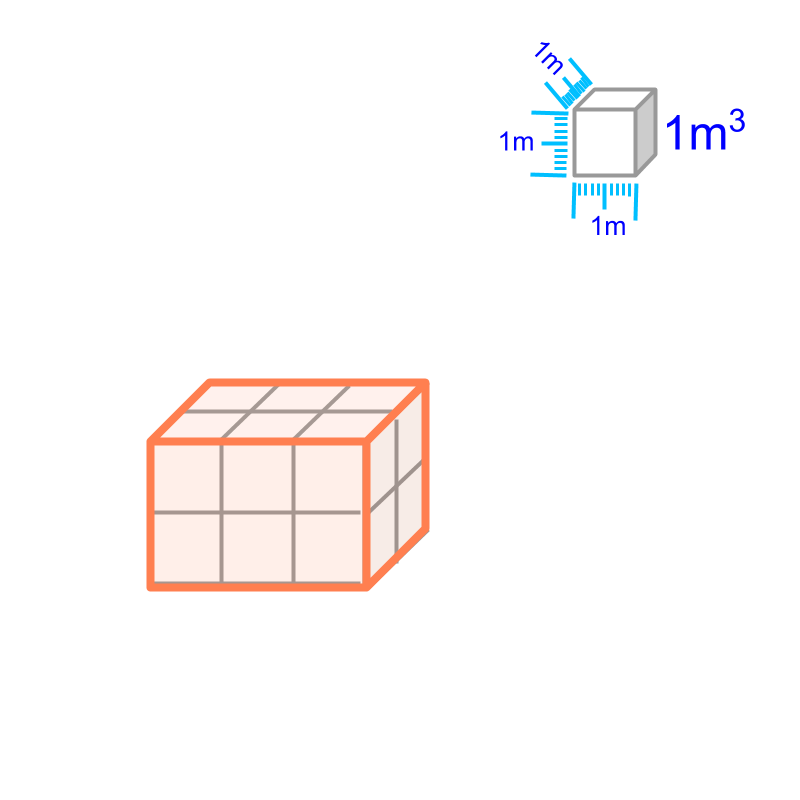maths > mensuration-basics

Introduction to Measuring Volume

what you'll learn...

Overview

Volume of a solid : The space-span of a solid is the volume of the solid. It is measured in cubic meter (or in one of other derived or similar forms.)Volume is specified as a number in reference to the space-span of a cube of $1$$1$ meter side.

space-span

Length of a rod is the distance-span measured in meter.

Area of a surface is the surface-span measured in square meter.

For a solid object that occupies space (like an apple, or a box), the measure of the space the volume of the object. Volume is the the space-span of a 3D-enclosed-solid.

To specify distance-span or length, a reference-prototype-standard (ie a metal rod of specific material at specific temperature) is used.

To specify surface-span or area, the area of a square with $1$$1$ meter sides is used as the reference.

Similarly, measurement of space-span is derived from the already defined measure for length.To specify the space-span or volume of a solid, a cube of side $1$$1$ meter is taken as the reference. The volume of that is one cubic meter or $1{m}^{3}$$1 {m}^{3}$. In the figure, the reference is shown at the top-right corner.

Volume of a solid is given in reference to the volume of a cube of side $1$$1$ meter. In the figure, the volume of the given cuboid is the number of $1$$1$ cubic meter cubes fit in that cuboid. It is counted to $6$$6$, so the volume of the cuboid is $6{m}^{3}$$6 {m}^{3}$.

The statement "volume of a box is $3$$3$ cubic meter" specifies that the space-span of the box equals $3$$3$ space-spans of a cube of side $1$$1$ meter

summary

Volume of a solid : The space-span of a solid is the volume of the solid. It is measured in cubic meter (or in one of other derived or similar forms.)Volume is specified as a number in reference to the space-span of a cube of $1$$1$ meter side.

Outline

The outline of material to learn "Mensuration basics : Length, Area, & Volume" is as follows.

•  Measuring Basics

→   Introduction to Standards

→   Measuring Length

→   Accurate & Approximate Meaures

→   Measuring Area

→   Measuring Volume

→   Conversion between Units of Measure

•  2D shapes

→   Perimeter of Polygons

→   Area of Square & rectangle

→   Area of Triangle

→   Area of Polygons

→   Perimeter and area of a Circle

→   Perimeter & Area of Quadrilaterals

•  3D shapes

→   Surface Area of Cube, Cuboid, Cylinder

→   Volume of Cube, Cuboid, Cylinder